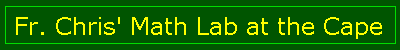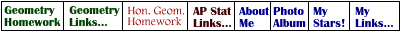Main Page

Lessons
Angles
Pool
Oil Pump
Basic Triangles
More Triangles
Art
Web Page
Euclid
Homework
Geometry
Hon Geometry

Web Calc
Pythagorean
Square Root
Fractions

Trivia
Impossibles
Trivia Quiz
Optical Quiz

TI Calc
Astronomy
Capuchins
FAQ

E-mail me!

# Ratios and the Fibonacci Sequence

The sequence 1,1,2,3,5,8,13,21,... is called a Fibonacci sequence named after its discoverer, Leonardo Fibonacci. He lived in the 13th century, like a famous saint you may have heard of.

The first two terms of the sequnce are 1 and 1. The idea is that the remaining terms are formed by adding the previous two terms. So the third term is 1+1=2, the next is 1+2=3, the next is 2+3=5, and so on..

The script in the web page computes the first few terms and calulates the ratio of the previous two terms.

 First Term (A) = Second Term (B) = Number of Terms to Compute (N) =

## Questions

1. Do you think the ratio will always be the same?

2. Press the "Compute" button and find out. Was your initial guess right?

3. As the terms become larger, what happens to the values of the ratios? (you may have to increase the number of terms (N) )

4. Suppose another sequence is formed by starting with different numbers for the the first and second term, and continuing in the same way as the Fibonacci sequence (That is, adding the previous two terms to get the next term). Do you think you will get a different ratio?

5. Set the first term (A) to "3" and the second term (B) to "11" and press the compute button again. Was the prediction you made in the previous question correct?

6. Try any two other numbers for the first and second term. What eventually happens to the ratio (you may have to increase the number of terms (N). (Try at least 30 terms.)

7. Compare this number to the Golden Ratio (press the Golden Ratio Button or look at page 253 in the Geometry text book. Do you see a connection? Is there any exceptions you can think of?
###### The javascript that makes this page work was written by Fr. Chris and adapted from page 261 of the Geometry Textbook. If you have any problems, comments or sugestions, please e-mail me at cct@ktb.net